Question

# Determine value of ΔH° for the oxidation of solid elemental sulfur to gaseous sulfur dioxide, S...

Determine value of ΔH° for the oxidation of solid elemental sulfur to gaseous sulfur dioxide, S (s, rhombic) + O2 (g) → SO2 (g)

For the reaction below, a) label the oxidation and reduction half reactions b) identify the substance oxidized and reduced c) identify the oxidizing and reducing agents Pb + PbO2 + 2H2SO4 → 2PbSO4 + 2H2O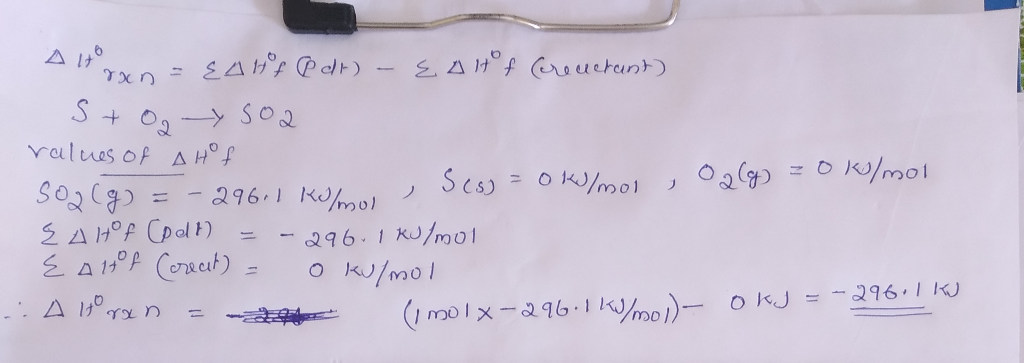#### Earn Coins

Coins can be redeemed for fabulous gifts.

Similar Homework Help Questions
• ### 16. Consider the combustion of solid sucrose (C12H22O11), to form liquid water and a gaseous product....

16. Consider the combustion of solid sucrose (C12H22O11), to form liquid water and a gaseous product. a) Write & balance the equation, including phases. b)If 2.001 g of sucrose reacts with 1.00 L of O2 at 753 torr and 25.8 °C, how many grams of liquid water are theoretically formed? c) Assign oxidation numbers to each element in the reaction. d) Which substance undergoes oxidation? Reduction? e) Identify the oxidizing and reducing agents.

• ### 1.) The value of S° for the oxidation of carbon to carbon dioxide, is __________ J/K...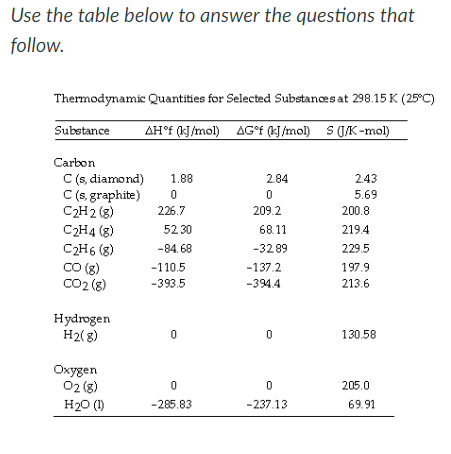1.) The value of S° for the oxidation of carbon to carbon dioxide, is __________ J/K mol. The combustion of carbon, as in charcoal briquettes, in the presence of abundant oxygen produces carbon dioxide. 2.)The value of S° for the reaction is __________ J/K. 3.)The value of S° for the decomposition of gaseous sulfur dioxide to solid elemental sulfur and gaseous oxygen, is __________ J/K mol. Use the table below to answer the questions that follow. Thermodynamic Quantities for Selected...

• ### 1. Provide the oxidation number for each of the underlined elements in following compounds or ions:...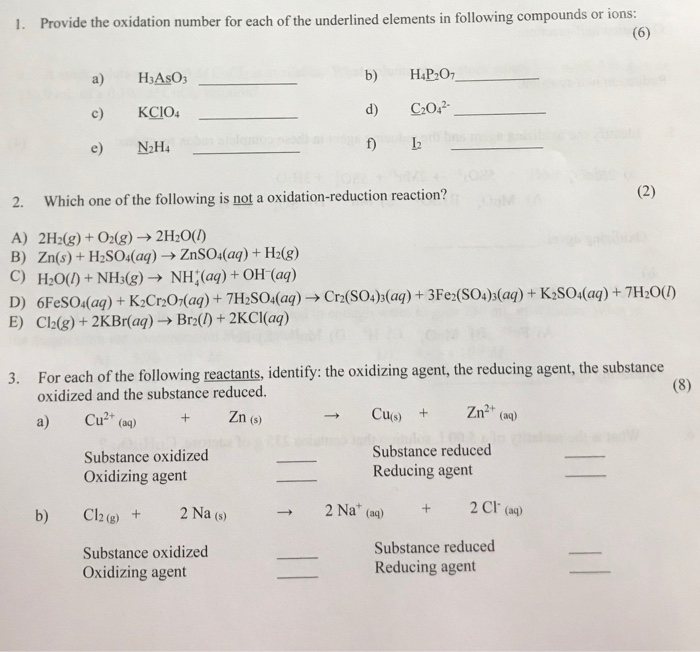1. Provide the oxidation number for each of the underlined elements in following compounds or ions: (6) a) H3AsO3 b) d) H4P207 C202² KCIO4 N2H4 2. Which one of the following is not a oxidation-reduction reaction? (2) A) 2H2(g) + O2(g) → 2H2O(1) B) Zn(s) + H2SO4(aq) → ZnSO4(aq) + H2(g) C) H2O(l) + NH3(g) → NH(aq) + OH(aq) D) FeSO4(aq) + K2Cr2OH(aq) + 7H2SO4(aq) → Cr2(SO4)3(aq) + 3Fe2(SO4)3(aq) + K2SO4(aq) + 7H2O(1) E) Cl2(g) +2KBr(aq) + Bra(l) + 2KCl(aq)...

• ### [References] Sc(s) + 3Ag+ (aq) + 3 Ag(s) + Sc3+ (aq) Redox? Oxidizing Agent Reducing Agent...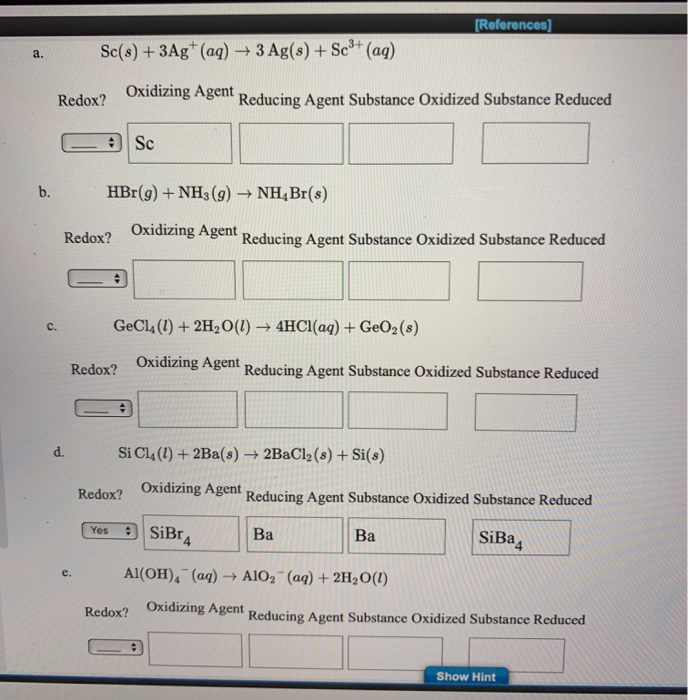[References] Sc(s) + 3Ag+ (aq) + 3 Ag(s) + Sc3+ (aq) Redox? Oxidizing Agent Reducing Agent Substance Oxidized Substance Reduced CE SC HBr(9) + NH3(g) + NH4Br(s) Redox? Oxidizing Agent in Reducing Agent Substance Oxidized Substance Reduced GeCl4 (1) + 2H2O(l) + 4HCl(aq) + GeO2 (s) Redox? Oxidizing Agent Reducing Agent Substance Oxidized Substance Reduced d. Si C14 (1) + 2Ba(s) + 2BaCl2(8) + Si(s) Redox? Oxidizing Agent Reducing Agent Substance Oxidized Substance Reduced Yes 2 SiBr, Ba SiBa Al(OH).-...

• ### This question has multiple parts. Work all the parts to get the most points. Specify which...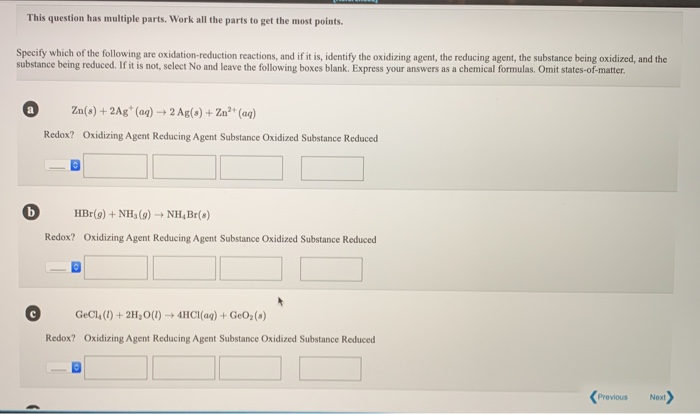This question has multiple parts. Work all the parts to get the most points. Specify which of the following are oxidation-reduction reactions, and if it is, identify the oxidizing agent, the reducing agent, the substance being oxidized, and the substance being reduced. If it is not, select No and leave the following boxes blank. Express your answers as a chemical formulas. Omit states-of-matter. Zn(s)+2Ag (aq)2 Ag(a) +Zn2+ (aq) Redox? Oxidizing Agent Reducing Agent Substance Oxidized Substance Reduced HBr(g) +NH, (g)NH4Br(s)...

• ### Gaseous sulfur dioxide, a component of acid rain, is made as a product of the combustion...

Gaseous sulfur dioxide, a component of acid rain, is made as a product of the combustion of sulfur-containing fuels. Calculate the volume of sulfur dioxide produced at 22.4 °C and 0.925 atm by the combustion of 8902 g of fuel that contains 5.55% of sulfur by mass. S8? (s) + 8 O2?(g)?? 8 SO2?(g)

• ### Chem 2A: Oxidation Numbers, Oxidation/Reduction rium 1. Determine the oxidation number of chlorine in the f...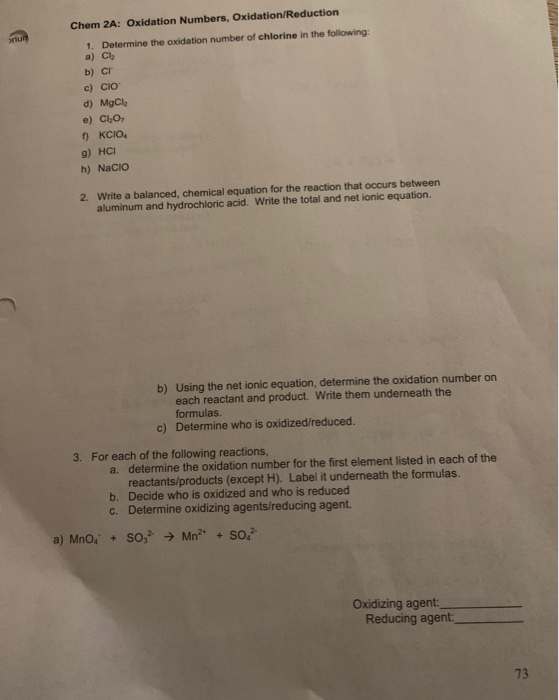Chem 2A: Oxidation Numbers, Oxidation/Reduction rium 1. Determine the oxidation number of chlorine in the following: a) Cl b) C c) CIO d) MgCl e) ClO ) KCIO g) HCI h) NaCIO 2. Write a balanced, chemical equation for the reaction that occurs between aluminum and hydrochloric acid. Write the total and net ionic equation. b) Using the net ionic equation, determine the oxidation number on each reactant and product. Write them underneath the formulas. Determine who is oxidized/reduced. c)...

• ### complete each of the following reactions and write the balanced molecular and net ionic equations for...

complete each of the following reactions and write the balanced molecular and net ionic equations for each reaction and identify the reaction type. For any redox reactions, show the oxidations numbers, identify the substance oxidized and the substance reduced, the oxidizing agent, and the reducing agent, and write the half reactions for oxidation and reduction. a. Lead II nitrate and sodium iodide react to form products b. Aluminum hydroxide is neutralized by sulfuric acid c. sulfur burns in oxygen to...

• ### (c) CH4(g) + 2 O2(g) → CO2(g) + 2 H2O(g) Is this an oxidation-reduction reaction? O...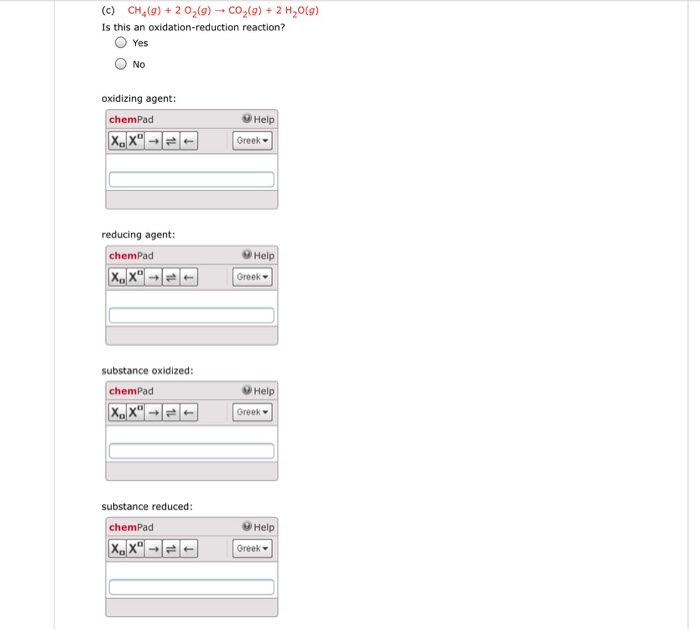(c) CH4(g) + 2 O2(g) → CO2(g) + 2 H2O(g) Is this an oxidation-reduction reaction? O Yes O No oxidizing agent: Help chemPad Greek- reducing agent: Help chemPad Greek ▼ substance oxidized: ⓤ Help chemPad Greek ▼ substance reduced: Help chemPad Greek▼

• ### Calculate the standard enthalpy of formation of gaseous sulfur dioxide (S02) using the following thermochemical information:...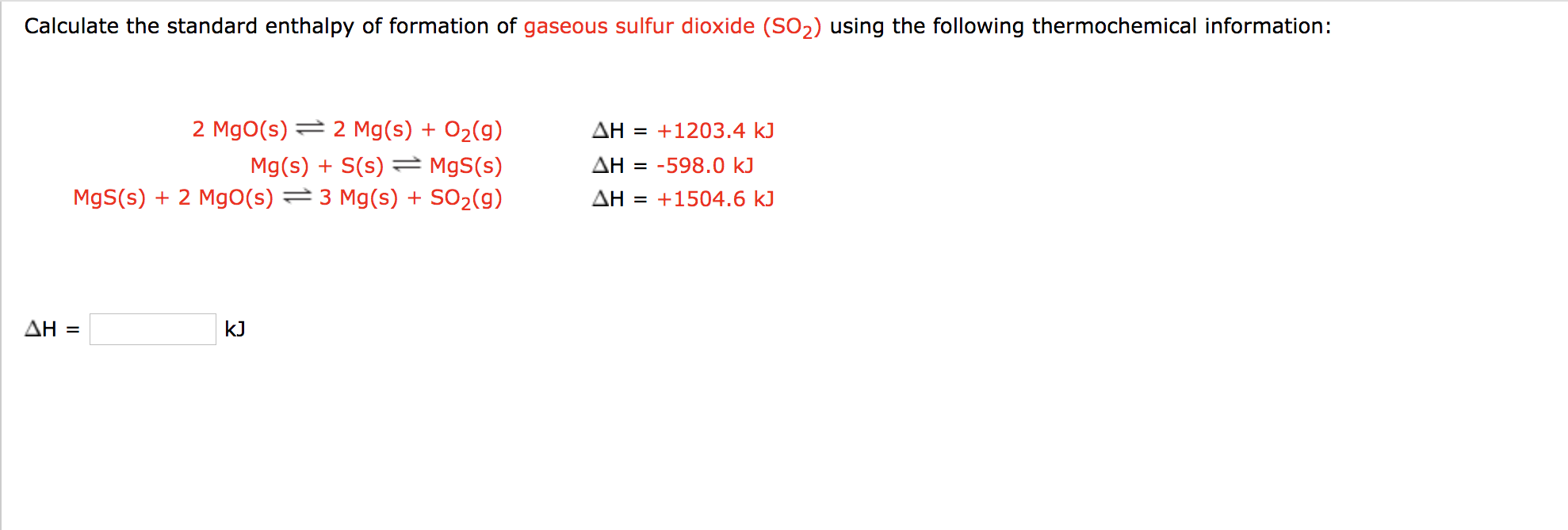Calculate the standard enthalpy of formation of gaseous sulfur dioxide (S02) using the following thermochemical information: 2 Mgo(s) = 2 Mg(s) + O2(g) Mg(s) + S(s) = MgS(s) Mgs(s) + 2 Mgo(s) = 3 Mg(s) + SO2(g) AH = +1203.4 k) AH = -598.0 kJ AH = +1504.6 kJ AH = k]